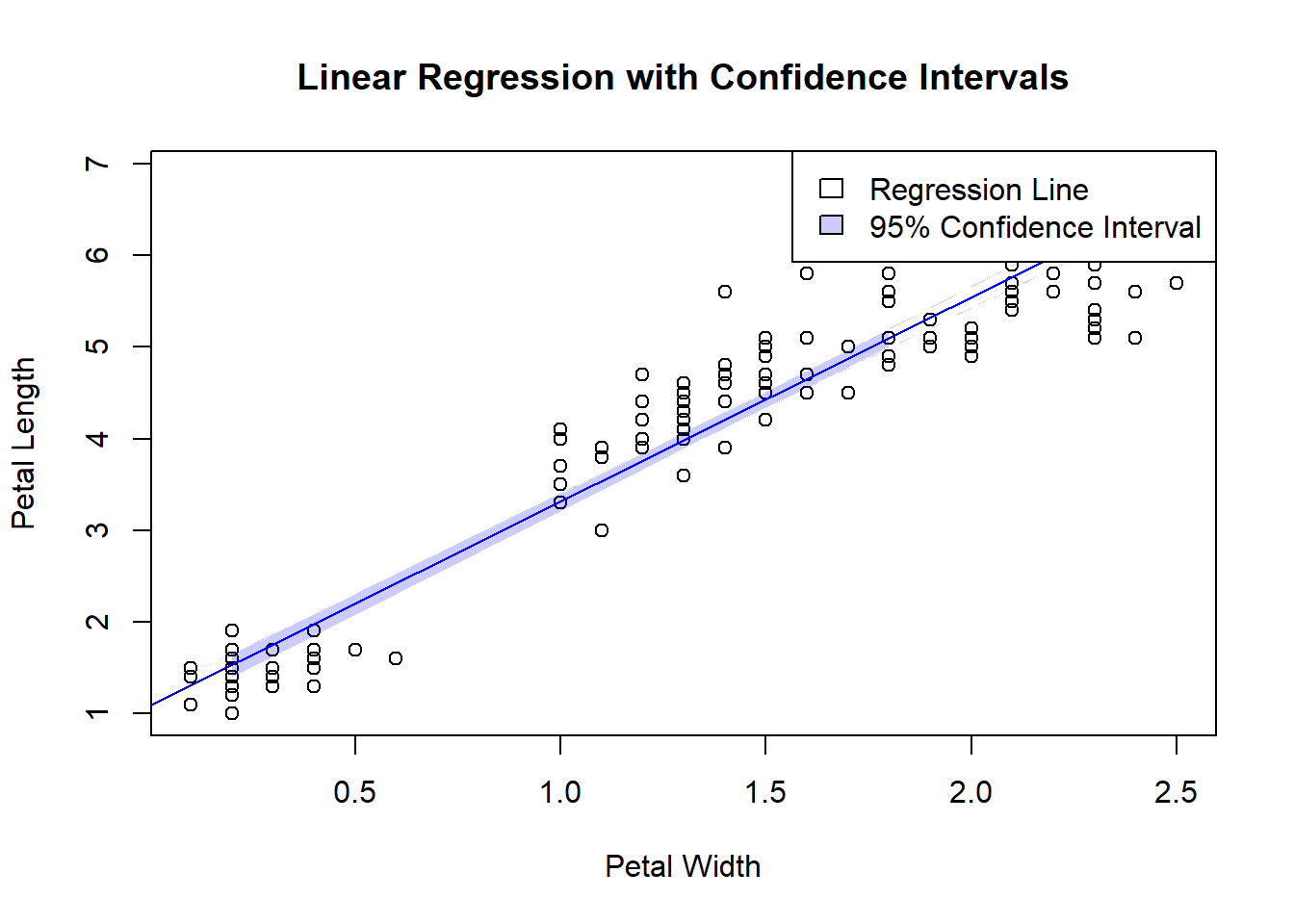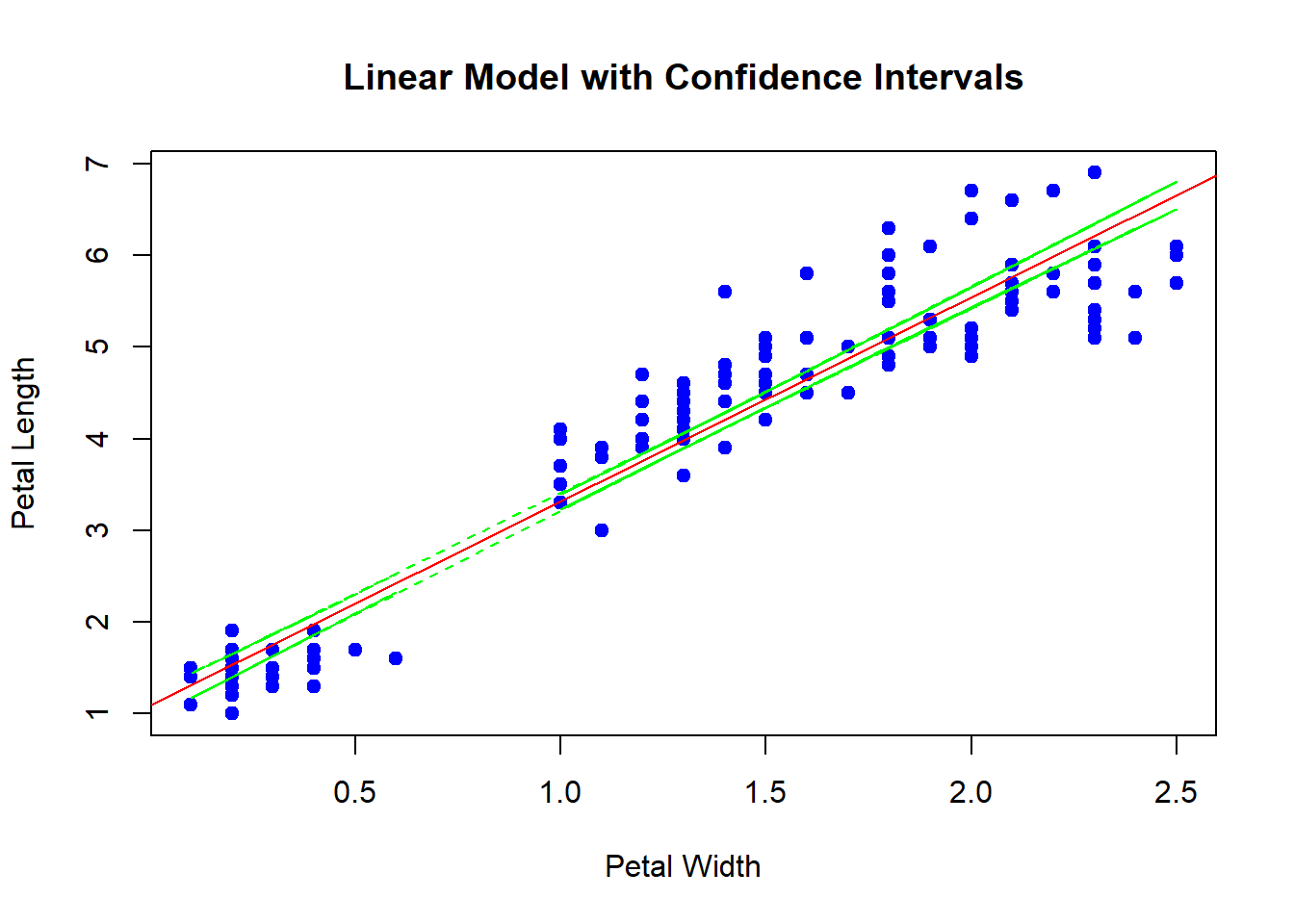# Creating Confidence Intervals for a Linear Model in R Using Base R and the Iris Dataset

rtip
viz
Author

Steven P. Sanderson II, MPH

Published

September 22, 2023

# Introduction

Linear regression is a fundamental statistical technique used to model the relationship between a dependent variable and one or more independent variables. While fitting a linear model is relatively straightforward in R, it’s also essential to understand the uncertainty associated with our model’s predictions. One way to visualize this uncertainty is by creating confidence intervals around the regression line. In this blog post, we’ll walk through how to perform linear regression and plot confidence intervals using base R with the popular Iris dataset.

The Iris dataset is a well-known dataset in the field of statistics and machine learning. It contains measurements of sepal length, sepal width, petal length, and petal width for three species of iris flowers: setosa, versicolor, and virginica. For our purposes, we’ll focus on predicting petal length based on petal width for one of the iris species.

First, let’s load the Iris dataset and take a quick look at its structure:

``````# Load the Iris dataset
data(iris)``````

Now view it

``````# View the first few rows of the dataset
``````  Sepal.Length Sepal.Width Petal.Length Petal.Width Species
1          5.1         3.5          1.4         0.2  setosa
2          4.9         3.0          1.4         0.2  setosa
3          4.7         3.2          1.3         0.2  setosa
4          4.6         3.1          1.5         0.2  setosa
5          5.0         3.6          1.4         0.2  setosa
6          5.4         3.9          1.7         0.4  setosa``````

# Fitting a Linear Model

We want to predict petal length (dependent variable) based on petal width (independent variable). To do this, we’ll fit a linear regression model using the `lm()` function in R:

``````# Fit a linear regression model
model <- lm(Petal.Length ~ Petal.Width, data = iris)``````

Now that we have our model, let’s move on to creating confidence intervals for the regression line.

# Calculating Confidence Intervals

To calculate confidence intervals for the regression line, we’ll use the `predict()` function with the `interval` argument set to “confidence”:

``````# Calculate confidence intervals
confidence_intervals <- predict(
model,
interval = "confidence",
level = 0.95
)

# View the first few rows of the confidence intervals
``````       fit      lwr      upr
1 1.529546 1.402050 1.657042
2 1.529546 1.402050 1.657042
3 1.529546 1.402050 1.657042
4 1.529546 1.402050 1.657042
5 1.529546 1.402050 1.657042
6 1.975534 1.863533 2.087536``````

The `confidence_intervals` object now contains the lower and upper bounds of the confidence intervals for our predictions.

# Creating the Plot

With the confidence intervals calculated, we can create a visually appealing plot to display our linear regression model and the associated confidence intervals:

``````# Create a scatterplot of the data
plot(
iris\$Petal.Width,
iris\$Petal.Length,
main = "Linear Regression with Confidence Intervals",
xlab = "Petal Width", ylab = "Petal Length"
)

abline(model, col = "blue")

polygon(
c(iris\$Petal.Width, rev(iris\$Petal.Width)),
c(
confidence_intervals[, "lwr"],
rev(confidence_intervals[, "upr"])
),
col = rgb(0, 0, 1, 0.2), border = NA)

legend(
"topright",
legend = c("Regression Line", "95% Confidence Interval"),
col = c("blue", rgb(0, 0, 1, 0.2)),
fill = c(NA, rgb(0, 0, 1, 0.2))
)``````In this plot, we start by creating a scatterplot of the data points, then overlay the regression line in blue. The shaded area represents the 95% confidence interval around the regression line, giving us an idea of the uncertainty in our predictions.

Here is a slightly different method, the confidence intervals:

``````# Calculate confidence intervals
conf_intervals <- predict(model, interval = "confidence")``````

Now the plot:

``````# Create a scatterplot
plot(
iris\$Petal.Width,
iris\$Petal.Length,
main = "Linear Model with Confidence Intervals",
xlab = "Petal Width",
ylab = "Petal Length",
pch = 19,
col = "blue"
)

abline(model, col = "red")

lines(
iris\$Petal.Width,
conf_intervals[, "lwr"],
col = "green",
lty = 2
)
lines(
iris\$Petal.Width,
conf_intervals[, "upr"],
col = "green",
lty = 2
)``````# Conclusion

In this blog post, we’ve demonstrated how to perform linear regression and plot confidence intervals using base R with the Iris dataset. Understanding and visualizing the uncertainty associated with our regression model is crucial for making informed decisions based on the model’s predictions. You can apply these techniques to other datasets and regression problems to gain deeper insights into your data.

Linear regression is just one of the many statistical techniques that R offers. As you continue your data analysis journey, you’ll find R to be a powerful tool for exploring, modeling, and visualizing data.